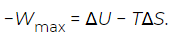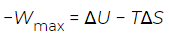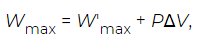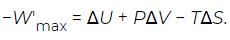Courses

# Thermodynamic potentials Physics Notes | EduRev

## Physics : Thermodynamic potentials Physics Notes | EduRev

The document Thermodynamic potentials Physics Notes | EduRev is a part of the Physics Course Basic Physics for IIT JAM.
All you need of Physics at this link: Physics

Most real thermodynamic systems are open systems that exchange heat and work with their environment, rather than the closed systems described thus far. For example, living systems are clearly able to achieve a local reduction in their entropy as they grow and develop; they create structures of greater internal energy (i.e., they lower entropy) out of the nutrients they absorb. This does not represent a violation of the second law of thermodynamics, because a living organism does not constitute a closed system.
In order to simplify the application of the laws of thermodynamics to open systems, parameters with the dimensions of energy, known as thermodynamic potentials, are introduced to describe the system. The resulting formulas are expressed in terms of the Helmholtz free energy and the Gibbs free energy G, named after the 19th-century German physiologist and physicist Hermann von Helmholtz and the contemporaneous American physicist Josiah Willard Gibbs. The key conceptual step is to separate a system from its heat reservoir. A system is thought of as being held at a constant temperature T by a heat reservoir (i.e., the environment), but the heat reservoir is no longer considered to be part of the system. Recall that the internal energy change (ΔU) of a system is given by
ΔU = Q − W,    (7)

Where Q is the heat absorbed and W is the work done. In general, Q and separately are not state functions, because they are path-dependent. However, if the path is specified to be any reversible isothermal process, then the heat associated with the maximum work (Wmax) is Qmax = TΔS. With this substitution the above equation can be rearranged as(8)

Note that here ΔS is the entropy change just of the system being held at constant temperature, such as a battery. Unlike the case of an isolated system as considered previously, it does not include the entropy change of the heat reservoir (i.e., the surroundings) required to keep the temperature constant. If this additional entropy change of the reservoir were included, the total entropy change would be zero, as in the case of an isolated system. Because the quantities UT, and S on the right-hand side are all state functions, it follows that −Wmax must also be a state function. This leads to the definition of the Helmholtz free energy
F = U − TS     (9)
such that, for any isothermal change of the system,
ΔF = ΔU − TΔS       (10)

Is the negative of the maximum work that can be extracted from the system. The actual work extracted could be smaller than the ideal maximum, or even zero, which implies that W ≤ −ΔF, with equality applying in the ideal limiting case of a reversible process. When the Helmholtz free energy reaches its minimum value, the system has reached its equilibrium state, and no further work can be extracted from it. Thus, the equilibrium condition of maximum entropy for isolated systems becomes the condition of minimum Helmholtz free energy for open systems held at constant temperature. The one additional precaution required is that work done against the atmosphere be included if the system expands or contracts in the course of the process being considered. Typically, processes are specified as taking place at constant volume and temperature in order that no correction is needed.
Although the Helmholtz free energy is useful in describing processes that take place inside a container with rigid walls, most processes in the real world take place under constant pressure rather than constant volume. For example, chemical reactions in an open test tube—or in the growth of a tomato in a garden—take place under conditions of (nearly) constant atmospheric pressure. It is for the description of these cases that the Gibbs free energy was introduced. As previously established, the quantity.(11)

Is a state function equal to the change in the Helmholtz free energy. Suppose that the process being considered involves a large change in volume (ΔV), such as happens when water boils to form steam. The work done by the expanding water vapour as it pushes back the surrounding air at pressure P is PΔV. This is the amount of work that is now split out from Wmax by writing it in the form(12)

where Wmax is the maximum work that can be extracted from the process taking place at constant temperature T and pressure P, other than the atmospheric work (PΔV). Substituting this partition into the above equation for −Wmax and moving the PΔV term to the right-hand side then yields(13)

This leads to the definition of the Gibbs free energy
G = U + PV − TS      (14)
such that, for any isothermal change of the system at constant pressure,
ΔG = ΔU + PΔV − TΔS      (15)

Is the negative of the maximum work Wmax that can be extracted from the system, other than atmospheric work. As before, the actual work extracted could be smaller than the ideal maximum, or even zero, which implies that W′ ≤ −ΔG, with equality applying in the ideal limiting case of a reversible process. As with the Helmholtz case, when the Gibbs free energy reaches its minimum value, the system has reached its equilibrium state, and no further work can be extracted from it. Thus, the equilibrium condition becomes the condition of minimum Gibbs free energy for open systems held at constant temperature and pressure, and the direction of spontaneous change is always toward a state of lower free energy for the system (like a ball rolling downhill into a valley). Notice in particular that the entropy can now spontaneously decrease (i.e., TΔS can be negative), provided that this decrease is more than offset by the ΔU + PΔV terms in the definition of ΔG. As further discussed below, a simple example is the spontaneous condensation of steam into water. Although the entropy of water is much less than the entropy of steam, the process occurs spontaneously provided that enough heat energy is taken away from the system to keep the temperature from rising as the steam condenses.
A familiar example of free energy changes is provided by an automobile battery. When the battery is fully charged, its Gibbs free energy is at a maximum, and when it is fully discharged (i.e., dead), its Gibbs free energy is at a minimum. The change between these two states is the maximum amount of electrical work that can be extracted from the battery at constant temperature and pressure. The amount of heat absorbed from the environment in order to keep the temperature of the battery constant (represented by the TΔS term) and any work done against the atmosphere (represented by the PΔV term) are automatically taken into account in the energy balance.

Offer running on EduRev: Apply code STAYHOME200 to get INR 200 off on our premium plan EduRev Infinity!

,

,

,

,

,

,

,

,

,

,

,

,

,

,

,

,

,

,

,

,

,

;